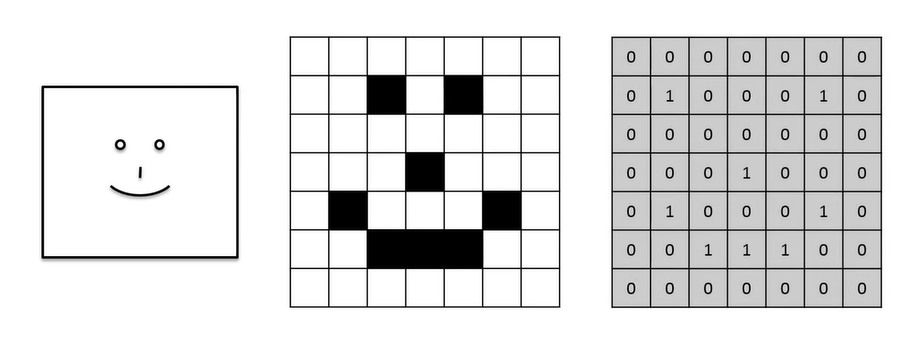# Convolution in Convolutional Neural Network(CNN)

Original article was published by Manik Soni on Artificial Intelligence on Medium# Convolution in Convolutional Neural Network(CNN)

## What is Convolution?

Convolution may be defined as a mathematical function which is given below.

It is a modification of 2 functions it shows how one function modifies the shape of another function.

## Let’s visualize What Convolution is?

Example: Let’s consider an emoji trained CNN which gives the description of the image what is the nature of an emoji(happy, sad,etc.).

So, in order to under the convolution, there are 3 elements that are taken into consideration:

1. Input image: The image that is sent to do the categorization.
2. Feature Detector: Mapping the image to the input image to extract some essential features. Like features that help to best describe an image.
3. Feature Map: Feature Map is a result of the extracted features. So consider this example where we take the image in an array format and we match the feature with the input image matrix

So consider this example where we take the image in an array format and we match the feature with the input image matrix. If the match happens when we add up the sum of the individual match.

In the above image, no match happen.

In the above image, you can see only one match happen.

So, repeat this process until the end.

So, you can see how we can detect individual features and create a feature map that helps to extract the essential feature in the input image, which helps to do the categorization.

In the real-life application, we have work on multiple feature maps called Convolutional Layer. Where individual feature contributes to give particular information.

Another application of Convolution is adding filters to images.

Filter 1: Sharpen is a filter that increases the pixel intensity.

Filter 2: Blur is a filter that fades the image density.

Filter 3: Edge Detect helps to detect the boundaries.

Other Filters: